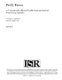## A Computationally Efficient Feasible Sequential Quadratic Programming Algorithm

 dc.contributor.advisor Tits, Andre en_US dc.contributor.author Lawrence, Craig T. en_US dc.contributor.department ISR en_US dc.date.accessioned 2007-05-23T10:06:10Z dc.date.available 2007-05-23T10:06:10Z dc.date.issued 1998 en_US dc.description.abstract The role of optimization in both engineering analysis and designis continually expanding. As such, faster and more powerful optimization algorithms are in constant demand. In this dissertation, motivated by problems from engineering analysis and design, new Sequential Quadratic Programming (SQP) algorithms generating feasible iterates are described and analyzed. What distinguishes these algorithms from previous feasible SQP algorithms is a dramatic reduction in the amount of computation required to generate a new iterate while still enjoying the same global and fast local convergence properties.

First, a basic algorithm which solves the standard smooth inequality constrained nonlinear programming problem is considered. The main idea involves a simple perturbation of the Quadratic Program (QP) for the standard SQP search direction. The perturbation has the property that a feasible direction is always obtained and fast local convergence is preserved. An extension of the basic algorithm is then proposed which solves the inequality constrained mini-max problem. The algorithm exploits the special structure of the problem and is shown to have the same global and local convergence properties as the basic algorithm.

Next, the algorithm is extended to efficiently solve problems with very many objective and/or constraint functions. Such problems often arise in engineering design as, e.g., discretized Semi-Infinite Programming (SIP) problems. The key feature of the extension is that only a small subset of the objectives and constraints are used to generate a search directionat each iteration. The result is much smaller QP sub-problems and fewer gradient evaluations.

The algorithms all have been implemented and tested. Preliminary numericalresults are very promising. The number of iterations and function evaluations required to converge to a solution are, on average, roughly the same as for a widely available state-of-the-art feasible SQP implementation, whereas the amount of computation required per iteration is much less. The ability of the algorithms to effectively solve real problems from engineering design is demonstrated by considering signal set design problems for optimal detection in the presence of non-Gaussian noise. en_US dc.format.extent 1390737 bytes dc.format.mimetype application/pdf dc.identifier.uri http://hdl.handle.net/1903/5971 dc.language.iso en_US en_US dc.relation.ispartofseries ISR; PhD 1998-5 en_US dc.subject algorithms en_US dc.subject computer aided design CAD en_US dc.subject optimization en_US dc.subject feasible iterates en_US dc.subject sequential quadratic programming en_US dc.subject superlinear convergence en_US dc.subject global convergence, en_US dc.title A Computationally Efficient Feasible Sequential Quadratic Programming Algorithm en_US dc.type Dissertation en_US

##### Original bundle
Now showing 1 - 1 of 1